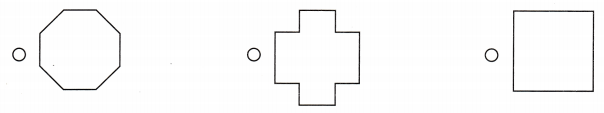# Texas Go Math Grade 2 Lesson 14.2 Answer Key More Two-Dimensional Shapes

Refer to our Texas Go Math Grade 2 Answer Key Pdf to score good marks in the exams. Test yourself by practicing the problems from Texas Go Math Grade 2 Lesson 14.2 Answer Key More Two-Dimensional Shapes.

## Texas Go Math Grade 2 Lesson 14.2 Answer Key More Two-Dimensional Shapes

Explore

Use a ruler. Draw the shape. Write the numbers.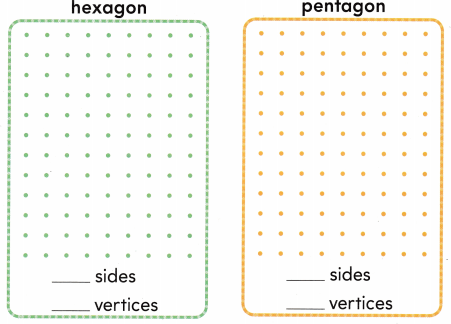FOR THE TEACHER • Read the following problem. Levi wants to draw a hexagon and a pentagon. What shapes could Levi draw? How many sides and vertices do each of Levis shapes have? Have children use rulers as straight edges for drawing the sides of the shapes.Explanation:
Levi drew a hexagon which has 6 sides and 6 vertices and a pentagon which has 5 sides and 5 vertices.

Math Talk
Mathematical Processes

Describe how the two shapes are the same and how they are different.
The two shapes are polygons and the number of sides and vetices of these two shapes are different.

Model and Draw

A polygon is any closed, two-dimensional shape with only straight sides.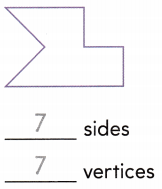An octagon has 8 sides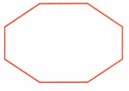__________ sides
__________ vertices
8 sides
8 vertices

Explanation:
The above shape has 8 sides and 8 vertices.__________ sides
__________ vertices
10 sides
10 vertices

Explanation:
The above shape has 10 sides and 10 vertices.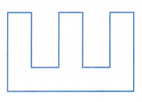__________ sides
__________ vertices
12 sides
12 vertices

Explanation:
The above shape has 12 sides and 12 vertices.

Share and Show

Write the number of sides and the number of vertices for each polygon.

Question 1.__________ sides
__________ vertices
9 sides
9 vertices

Explanation:
The above shape has 9 sides and 9 vertices.

Question 2.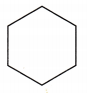__________ sides
__________ vertices
6 sides
6 vertices

Explanation:
The above shape has 6 sides and 6 vertices.

Question 3.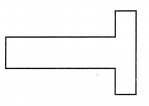__________ sides
__________ vertices
8 sides
8 vertices

Explanation:
The above shape has 8 sides and 8 vertices.

Problem Solving

Write the number of sides and the number of vertices for each polygon.

Question 4.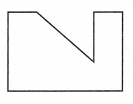__________ sides
__________ vertices
7 sides
7 vertices

Explanation:
The above shape has 7 sides and 7 vertices.

Question 5.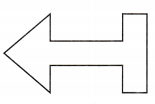__________ sides
__________ vertices
11 sides
11 vertices

Explanation:
The above shape has 11 sides and 11 vertices.

Question 6.
H.O.T. Kelt drew these three shapes. She says they are all polygons. Is Kat correct? Explain.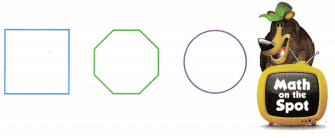Explanation:
No Kelt is wrong as the shapes that are circled are only polygons but the shape circle is not a polygon.

Question 7.
H.O.T. Multi-Step Tim wants to draw a polygon with more than 9 sides but fewer than 13 sides. Draw more sides to show a polygon Tim could draw.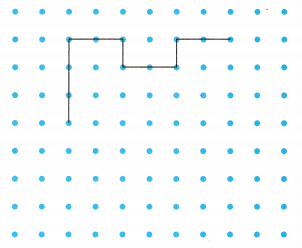The polygon has __________ sides.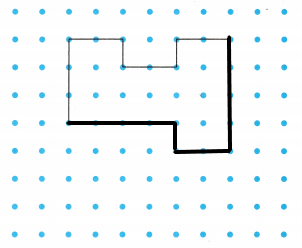Explanation:
I drew the leftover polygon.The polygon above has 10 sides.Question 8.
Use Diagrams Which shape has exactly 10 sides?
(A) B
(B) C
(C) A
Shape (C) has exactly 10 sides.

Question 9.
Which shape has exactly 8 vertices?
(A) D
(B) A
(C) C
Shape (B) has exactly 8 vertices.

Question 10.
Multi-Step Which shapes have fewer than 10 sides?
(A) A and B
(B) C and D
(C) B and D
Shapes B and D have fewer than 10 sides.

Question 11.
Texas Test Prep How many sides does this polygon have?(A) 11 sides
(B) 12 sides
(C) 8 sides
12 sides

Explanation:
The above shape has 12 sides.

TAKE HOME ACTIVITY • Hove your child explain how he or she solved one of the problems in this lesson.

### Texas Go Math Grade 2 Lesson 14.2 Homework and Practice Answer Key

Write the number of sides and the number of vertices for each polygon.

Question 1.__________ sides
__________ vertices
6 sides
6 vertices

Explanation:
The above shape has 6 sides and 6 vertices.

Question 2.__________ sides
__________ vertices
9 sides
9 vertices

Explanation:
The above shape has 9 sides and 9 vertices.

Problem Solving

Question 3.
Cheryl drew these three shapes. She says they are all polygons. Is Cheryl correct? Explain.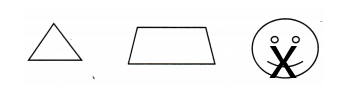Explanation:
Cheryl is wrong as the shape that is marked X is not a polygon as it donot have any sides.

Question 4.
Multi-Step Ethan starts to draw a polygon that looks like a house. Draw more sides for the polygon. How many sides does the polygon have?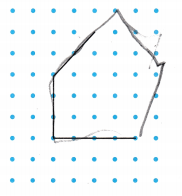The polygon has __________ sides.
The polygon has 5 sides.

Lesson Check

Question 5.
Which shape has more than 10 sides?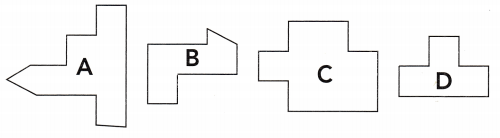(A) C
(B) B
(C) A
(C)

Explanation:
The shape A has more than 10 sides.

Question 6.
How many sides does a hexagon have?
(A) 8
(B) 6
(C) 10
(B) 6

Explanation:
A hexagon has 6 sides.

Question 7.
Which shape is a pentagon?Explanation:
A pentagon has 5 sides.I marked the shape that has 5 sides.

Question 8.
Which shape is an octagon?